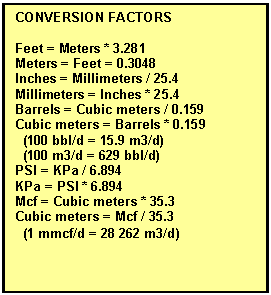Productivity Index
Estimating productivity is a useful way to compare the quality of wells from similar reservoirs, even when results are not calibrated. Calibration to core permeability is practical in most sands and shaly sands. Carbonate reservoirs are more heterogeneous and calibration of log analysis productivity estimates is not usually possible. Pressure transient analysis to estimate flow capacity, KH, is useful for all reservoirs, and the equations below offer a quick look method to solve for productivity from any source. These are simplified versions of the equations, and reservoir engineers may use more exact solutions.

STEP 1: Productivity index

1: Qo = KV1 * Kh * (PF – PS) / VISO

2: Qg = KV2 * Kh * ((PF – PS) ^ 2) / (FT + KT2)

Where:  KV1 = 0.0001 for English units (bbl/day)

KV1 = 0.000 007 560 for Metric units (m3/d)

KV2 = 0.0001 for English units (mcf/day)

KV2 = 0.000 010 920 for Metric units (m3/d)

KT2 = 460 'F for English units

KT2 = 273 'C for Metric units

Results are in bbl/day or m3/day for oil and mcf/d or e3m3/day for gas. The estimated flow rate (Qo or Qg) is called the initial deliverability, Qd. Wells are often rate limited by law or facilities to an initial rate, Qi, which is less than deliverability, Qd.

Where:
BHT = bottom hole temperature (degrees F or degrees C)
BHTDEP = depth of bottom hole temperature (ft or m)
DEPTH = average formation depth (ft or m)
Kh = flow capacity or permeability-thickness product (md-ft or md-m)
PFa = average formation pressure (psi or KPa)PSa = average surface or pipeline delivery pressure (psi or KPa)
SUFT = actual surface (degrees F or degrees C)
TFa = formation temperature, absolute (degrees F or degrees C)
Qg = productivity gas (mcf/day or m3/day)
Qo = productivity oil (bbl/day or m3/day)
VISO = oil viscosity (cp)COMMENTS:
These formulae are "log analysis" versions of more complex formulae should be used as guides to well quality only. Any resemblance to absolute open flow data or real well tests is coincidental. It is most useful for comparing the potential of two or more zones to help decide which one to complete first. The highest Qo will probably earn the most money or at least return it soonest.RECOMMENDED PARAMETERS:
NoneNUMERICAL EXAMPLE:
1. Assume data for Sand B at 1000 meters (3000 feet):
P = 1800 psi
VISO = 1.0 cp
FT = 160 degrees Fahrenheit
THICK = 15 feet
Perm = 670 md
Kh = 15 * 679 = 10185 md-ft

If oil well:
Qo = 0.1 * 10185 * 1800 / (1000 * 1.0) = 1833 bopd

If gas well:
Qg = 0.0001 * 10185 * (1800 ^ 2) / (160 + 460) = 5322 mcf/day

Page Views ---- Since 01 Jan 2015
Copyright 2023 by Accessible Petrophysics Ltd.
CPH Logo, "CPH", "CPH Gold Member", "CPH Platinum Member", "Crain's Rules", "Meta/Log", "Computer-Ready-Math", "Petro/Fusion Scripts" are Trademarks of the Author